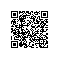# 阿里云服务器宽带选多少合适？

1M宽带
128KB/秒

2M宽带
2128=256KB/秒

3M宽带
3
128=384KB/秒

4M宽带
4128=512KB/秒

5M宽带
5
128=640KB/秒

6M宽带
6128=768KB/秒

7M宽带
7
128=896KB/秒

8M宽带
8128=1024KB/S=1M/秒

9M宽带
9
128=1152KB/秒=1.125M/秒

10M宽带
10128=1280KB/秒=1.25M/秒

20M宽带
20
128=2560KB/秒=2.5M/秒

50兆宽带
50128=6400KB/秒=6.25M/秒

100兆宽带
100
128=12800KB/秒=12.5M/秒

200兆宽带
200128=25600KB/秒=25M/秒

N兆宽带
N
128，以此类推

# 注意阿里云5M宽带分割点

## 保守选择宽带使用钉钉扫一扫加入圈子
+ 订阅